## Fun with Integer Sequences and SAS

Started ‎05-19-2023 by
Modified ‎05-19-2023 by
Views 998
SAS OnDemand for Academics has replaced SAS University Edition as a free e-learning option. Hit the orange button below to start your journey with SAS OnDemand for Academics:

We’ve all seen those number puzzles where you are given a sequence of integers and have to work out the next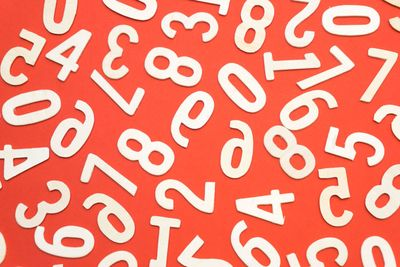number in the sequence. Once you’ve got the hang of them, they tend to be pretty straightforward, involving adding some of the previous numbers in the sequence, possibly skipping one or more. There are, however, much more complex ways of generating sometimes interesting and useful sequences. In this edition of Free Data Friday, we will be looking at The Online Encyclopaedia of Integer Sequences which holds data for over a quarter of a million integer sequences of varying complexity and usefulness. We will see how you can use SAS to create custom functions to generate the nth number in a sequence. Even where there is no immediate practical value to a sequence, they can be useful for practicing your programming techniques, setting problems for trainees or even code golf type puzzles for the more experienced, so don’t dismiss any sequence out of hand.

## Get the data

There’s no real data which needs to be downloaded here. Instead, each encyclopaedia entry consists of a web page giving the first few numbers of a sequence along with a description, formula for calculating the sequence, cross references to other sequences, programs for generating the sequence in mathematica and other languages (but not SAS) and other information. You can also search for a particular sequence or request a random sequence to be displayed.

## Get started with SAS OnDemand for Academics

In this 9-minute tutorial, SAS instructor @DomWeatherspoon shows you how to get your data into SAS OnDemand for Academics and other key steps:

## Examples

We’re going to choose two sequences to show the process of creating custom SAS functions to generate the nth number in each sequence.

### Triangular Numbers

A triangular number is a number that can be represented by a pattern of dots arranged in an equilateral triangle. For example, 1, 3, 6 and 10 are all triangular numbers.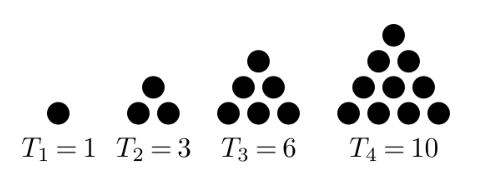We can use the following code to create a function which calculates a triangular number.

``````proc fcmp outlib=work.maths.intseqs;
function gettriangular(n);
retval=n*(n+1)/2;
return(retval);
endsub;
run;

options cmplib=work.maths;

data triangular;
do i=0 to 53;
triangular=gettriangular(i);
output;
end;
run;``````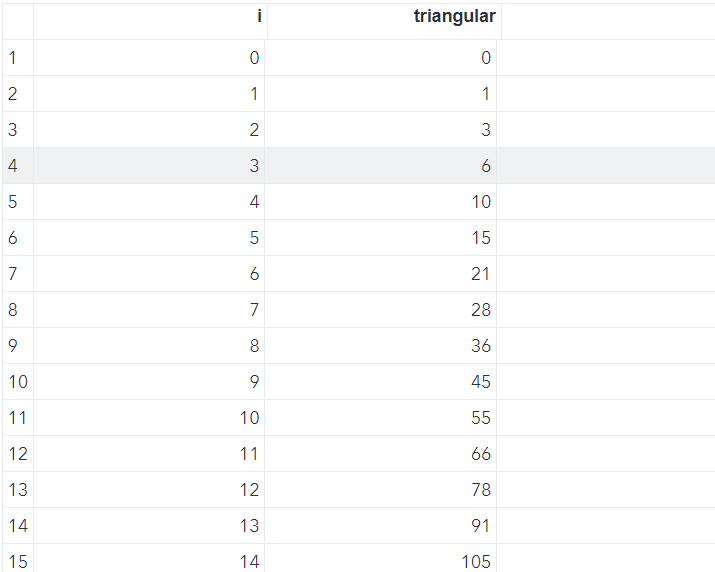As you can see the data step generates the first 54 triangular numbers (starting at zero). You can check the output against the list supplied in the encyclopaedia.

We are using the  FCMP Procedure with the outlib option. This saves the function to the specified library, dataset and package removing the need to regenerate the function every time you need to use it if you save it to a permanent library (as here I usually save to the temporary work library when I am developing functions). However, I strongly recommend saving the source code in case the dataset gets lost or accidentally deleted.

Triangular numbers have quite a few different uses including solving “handshake problems” e.g. In a room of x number of people how many unique handshakes must take place before everyone is introduced to everyone else?

Padovan numbers are defined by the following sequence and have applications in number theory and architecture.

P(0)=1

P(1)=0

P(2)=0

Thereafter

P(n)=P(n-3)+P(n-2)

This is much more complex to program as in order to calculate a number in the sequence beyond P(2) we need to know two other numbers in the sequence, which are themselves also Padovan numbers. We could, of course, calculate the full sequence of numbers every time we run the function but that would be very inefficient. Instead we will store all previously calculated numbers in a hash object and check to see if we have already calculated the required numbers. If we have, we will use them, if not then the function will call itself in a process known as recursion.

Here is the code (I've tried to make it and the comments as easy to follow as possible).

``````proc fcmp outlib=work.maths.intseqs;

/* 		Create a hash table - this persists for the life of */
/* 		the data step in which it will be called. It will */
/* 		NOT be recreated on subsequent calls within the */
/* 		data step */

declare hash h();

rc=h.definekey("seqno");
rc=h.definedone();

/* 		Set the first 3 padovan numbers */
seqno=n;
if n=0 then do;
notfound=h.check();
if notfound ne 0 then do;
end;
end;
else if n=1 then do;
notfound=h.check();
if notfound ne 0 then do;
end;
end;
else if n=2 then do;
notfound=h.check();
if notfound ne 0 then do;
end;
end;

/* 		Calculate subsequent padovan numbers		 */
else do;
seqno=n-2;
notfound=h.find();
if notfound ne 0 then do;
/* 		The Function calls itself recursively */
end;
seqno=n-3;
notfound=h.find();
if notfound ne 0 then do;
/* 		The Function calls itself recursively */
end;
retval=prev+prevprev;
end;
num=h.num_items();

return(retval);

endsub;

run;

options cmplib=work.maths;

/* Calculate a single Padovan Number */
i=30;
run;

/* Calculate a sequence of Padovan numbers */
data test;
do i=0 to 60;

output;

end;

run;``````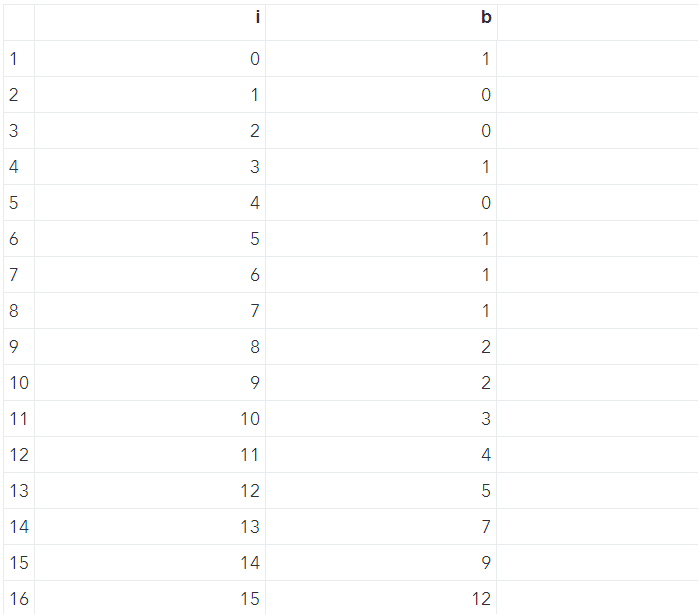There are two things to note:

1. Hash objects in an FCMP function behave slightly differently to “normal” hash objects. In particular they persist for the life of the data step they are being used in. The object is only created once and not every time the declare function is encountered; and
2. The function calls itself in a process known as recursion. It is vital that in recursive functions there is a guaranteed way out of the recursive loop otherwise the program can fall into a “black hole” of infinitely calling itself, running out of memory and crashing horribly. In our function the guaranteed way out is when the parameter n is less than 3, if not earlier.

Again, you can check the output from the list in the encyclopaedia.

Did you find a better way to generate these sequences or another really interesting sequence in the encyclopaedia? Share in the comments. I’m glad to answer any questions.

Hit the orange button below to see all the Free Data Friday articles.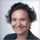Thanks for sharing the On-Line Encyclopedia of Integer Sequences. What an awesome site and also celebrating it's 50th year! I found this article which has some interesting history too https://link.springer.com/article/10.1007/s00283-023-10266-6

Interesting use of recursion with the Padovan numbers!

Cheers,

MichelleI would go with "static arrays" for this task, shorter code, easier to understand, and maintain, and I think faster.

``````proc fcmp outlib=work.maths.intseqs;

ARRAY P/NOSYMBOLS;
ARRAY T/NOSYMBOLS;
STATIC P T v 0;

/* initiate base */
if v=0 then
do;
p=1; /*P0*/
p=0; /*P1*/
p=0; /*P2*/
v=1;
end;

/* since arrays are 1 based we need to do n+1 */
if n+1 > dim(p) then
do;
/* extend temporary T to proper dimension */
call dynamic_array(T,n+1);
/* copy already calculated values */
do i = 1 to dim(P);
t[I]=p[i];
end;
/* calculate new values */
do i = i to n+1;
t[I]=t[i-3]+t[i-2];
end;
/* assign P with calculated result */
call dynamic_array(P,n+1);
do i = 1 to dim(P);
p[I]=t[i];
end;
end;
/* result */
return(P[n+1]);

endsub;

run;

options cmplib=work.maths;

/* Calculate a single Padovan Number */
i=30;
run;
proc print;
run;

/* Calculate a sequence of Padovan numbers */
data test;
do i=0 to 60, 20 to 0 by -1;
output;
end;
run;
proc print;
run;``````

BartIt is like a Fibonacci numbers . Here is my code just for fun.

``````data _null_;
array p{0:40} _temporary_;
p{0}=1;
p{1}=0;
p{2}=0;
do i=3 to 40;
p{i}=p{i-3}+p{i-2};
end;
do i=0 to 40;
put p{i}=;
end;
run;``````
```p=1
p=0
p=0
p=1
p=0
p=1
p=1
p=1
p=2
p=2
p=3
p=4
p=5
p=7
p=9
p=12
p=16
p=21
p=28
p=37
p=49
p=65
p=86
p=114
p=151
p=200
p=265
p=351
p=465
p=616
p=816
p=1081
p=1432
p=1897
p=2513
p=3329
p=4410
p=5842
p=7739
p=10252
p=13581
```@Ksharp - exactly ! 🙂

without _temporary_

``````data _null_;
array p{0:40};
p{0}=1;
p{1}=0;
p{2}=0;
do i=3 to hbound(p);
p{i}=p{i-3}+p{i-2};
end;

put (p{*}) (=/);
run;``````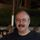Thanks @MichelleHomes that's a really interesting article. The encyclopaedia can get quite addictive browsing through the sequences and trying to figure out how to code them in SAS!

Also it's great to see the alternative versions from @yabwon and @Ksharp - I'd have to agree that arrays are probably simpler, particularly if you're not overly familiar with hash tables. I've done some simple testing of the two different methods with increasing numbers of padovan numbers calculated with the following results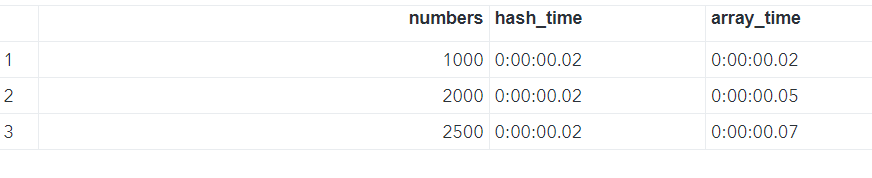The timings for the hash table version seem to be constant upto 2,500 calls but the array version times start to rise at some point over 1,000 where the two methods are neck and neck. If you try to calculate more than about 2,500 numbers you get an overflow error with both versions, which I was sort of expecting. I'd therefore say that the hash table method has a tiny theoretical advantage in speed on calculating a long sequence but it's not really noticeable in practice.Is the goal to make a FUNCTION or make the SERIES?  Much easier to create the SERIES as you can just use a DO loop.

For example for the PADOVAN series

``````
do n=0 to 60;
a=sum(a2,a3);
if n=0 then a=1;
else if n in (1,2) then a=0;
output;
a3=a2;
a2=a1;
a1=a;
end;
keep n a ;
run;
``````

But for the Triangle series there is no need to make a NEW function.

Just use the existing COMB() function.

``````data triangular;
do i=0 to 53;
triangular=comb(i+2,2);
output;
end;
run;``````

From the Encyclopedia entry

Number of legal ways to insert a pair of parentheses in a string of n letters. E.g., there are 6 ways for three letters: (a)bc, (ab)c, (abc), a(b)c, a(bc), ab(c). Proof: there are C(n+2,2) ways to choose where the parentheses might go, but n + 1 of them are illegal because the parentheses are adjacent. Cf. A002415.Thanks @Tom you're right using the SAS function comb(n,2) does generate triangular numbers and I'd missed that. The only difference is that for values less than 2 the comb function returns a missing value whereas mine returns 1 where n=0 and 0 where n=1 which is in line with the encyclopaedia.

To answer your other question I wanted to create a function which would return the nth number in a sequence. As you say it's much easier to generate full sequence every time but I wanted to show how you could do quite complex things with Proc FCMP and of course it's more effient to only generate the number(s) you need.

Version history
Last update:
‎05-19-2023 09:08 AM
Updated by:Contributors
Article Labels
Article Tags